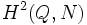# Supergroups of direct product of Z4 and Z2

## Contents

This article gives specific information, namely, supergroups, about a particular group, namely: direct product of Z4 and Z2.
View supergroups of particular groups | View other specific information about direct product of Z4 and Z2

This article discusses the possible supergroups of the direct product of Z4 and Z2, i.e., the abelian group of order eight that is a direct product of the cyclic group of order four and the cyclic group of order two.

## Classification of groups containing it as a subgroup of index two

Note that any subgroup of index two is normal.

### The general procedure

Suppose$G$ is a group of order sixteen containing a subgroup$N$ of order eight isomorphic to the direct product of Z4 and Z2. Note that since$N$ is of index two, it is a normal subgroup. Further, the quotient group is isomorphic to$Q$, the cyclic group of order two.

The classification has two steps:

• Find all homomorphisms from$Q$ to$\operatorname{Aut}(N)$.
• For each such homomorphism, find all possible extensions. These are classified by the elements of$H^2(Q,N)$ for the action.

### The classification$\operatorname{Aut}(N)$ is isomorphic to the dihedral group of order eight. There are five subgroups of order two in this group, coming in two conjugacy classes of size two each and one normal subgroup of order two

Thus, there are four equivalence classes of homomorphisms from$Q$ to$\operatorname{Aut}(N)$: the trivial map, the map with image equal to the normal subgroup of order two, the map with image equal to the subgroup in one of the conjugacy classes, and the map with image equal to a subgroup in the other conjugacy class.

Corresponding to the trivial map, we have the following extensions corresponding to elements of$H^2(Q,N)$:

• Direct product of Z4 and V4: This is a direct product of the cyclic group of order four and two copies of the cyclic group of order two. Specifically, it is a direct product of$N$ and a cyclic group of order two. This corresponds to the identity element of$H^2(Q,N)$.
• Direct product of Z8 and Z2
• Direct product of Z4 and Z4

For the map to the subgroup of order two generated by the inverse map (which is the normal subgroup of the automorphism group), we have the following extensions corresponding to elements of$H^2(Q,N)$, which has order two:

• Direct product of D8 and Z2: This is obtained as the semidirect product of$N$ by a group of order two acting by the inverse map. (Hence, this is also the generalized dihedral group). This corresponds to the identity element of$H^2(Q,N)$.
• Direct product of Q8 and Z2: This corresponds to the non-identity element of$H^2(Q,N)$.

For the map to one of the other two conjugacy classes, we have the groups:

For the map to the other conjugacy class, we have the groups: